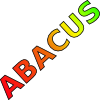#### You may also like### Consecutive Numbers

An investigation involving adding and subtracting sets of consecutive numbers. Lots to find out, lots to explore.### Tea Cups

Place the 16 different combinations of cup/saucer in this 4 by 4 arrangement so that no row or column contains more than one cup or saucer of the same colour.### Counting on Letters

The letters of the word ABACUS have been arranged in the shape of a triangle. How many different ways can you find to read the word ABACUS from this triangular pattern?

##### Age 11 to 14Challenge Level

Mr Skipper (who didn't send us his first name) was the first to send in a solution:

Wherever we place the square, the number in the top left corner is the smallest.

Call this $n$.

The arbitrary square becomes

n n+1 n+2

n+1 n+2 n+3

n+2 n+3 n+4

So the product of the top left and bottom right is

n(n+4) = n^2 + 4n.

The product of the top right and bottom left is

(n+2)^2 = n^2 + 4n + 4.

(n^2 + 4n + 4) - (n^2 + 4n) = 4.

So the difference is always 4.

Annette aged 13 did really well and generalised the problem still further. She considered what would happen if you had any sized square on the same addition grid.

Annette talks about the size of the square to be N by N, and the first number in the top left of the square to be k.

For an N x N square, the difference is -(N-1)*(N-1),

since k=number in top left then

k(k+2N-2)-(k+N-1)*(k+N-1)= -(N-1)*(N-1),